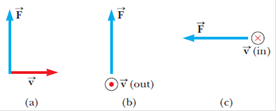# In Figure P19.3 assume in each case the velocity vector shown is replaced with a wire carrying a current in the direction of the velocity vector. For each case, find the direction of the magnetic field that will produce the magnetic force shown.FIGURE P19.3 replace the velocity vector with a current in that direction.

Question

In Figure P19.3 assume in each case the velocity vector shown is replaced with a wire carrying a current in the direction of the velocity vector. For each case, find the direction of the magnetic field that will produce the magnetic force shown.

FIGURE P19.3 replace the velocity vector with a current in that direction.